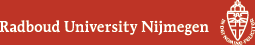## Geometry Seminar - Abstracts

### Talk

Thursday 10 November, 14:00 - 15:00 in HG03.085
Nikolai Tyurin (MPIM Bonn)
Special Bohr - Sommerfeld geometry

### Abstract

The main interest is the Lagrangian geometry of compact algebraic varieties. Every 1 - connected algebraic variety $X$ can be regarded as a real symplectic manifold if we choose an appropriate principal polarization, an ample line bundle. The particular choice of the corresponding Kahler form $\omega$ regarded as symplectic form is not important since all the forms from the same cohomology class are equivalent thus all the corresponding "Lagrangian geometries" are equivalent as well. The term "Lagrangian geometry" means that we study lagrangian submanifolds on $(X, \omega)$ so such real $S \subset X$ that $dim_{\mathbb{R}}S = dim_{\mathbb{C}} X$ and $\omega|_S \equiv 0$. The main aim is to construct certain finite dimensional moduli space of lagrangian submanifolds (or cycles = submanifolds with singularities of prescribed types) for algebraic varieties.

As examples: SpLag geometry proposed by N. Hitchin for Calabi - Yau varieties; ALAG moduli space of Bohr - Sommerfeld lagrangian cycles constructed by A. Tyurin and A. Gorodentsev.

Our way: Special Bohr - Sommerfeld submanifolds = certain mixed of SpLag - and BS- approaches, applicable for much more wider situation than CY, in general for any compact 1 - connected algebraic variety.

(Back to geometry seminar schedule)